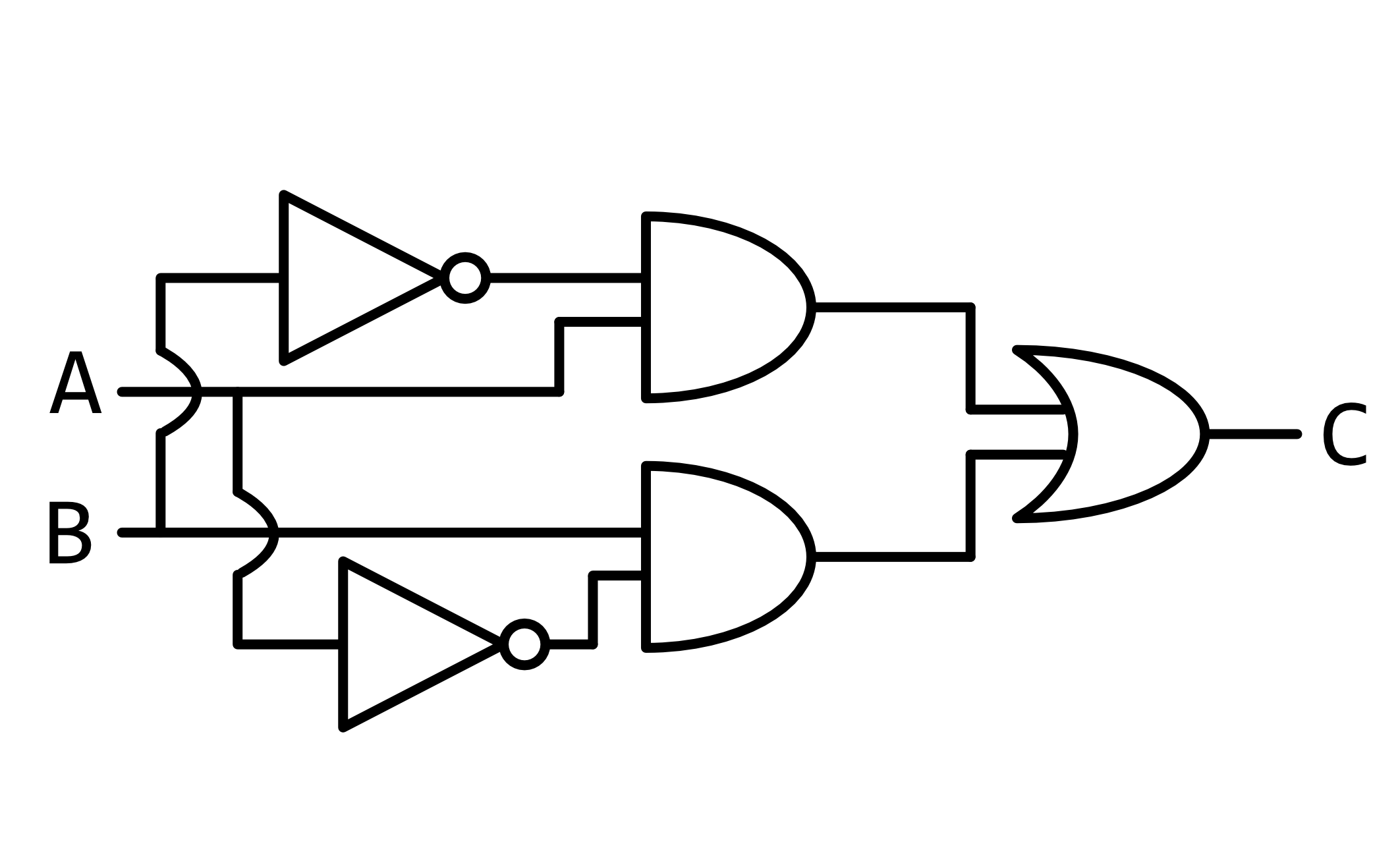# Logical Questions

Even though their syntax differs, Scratch, C, Python, and SQL all have “logical operators” via which you can express “and”, “or”, and “not”, each of which evaluates to a Boolean value, “true” or “false”.

It turns out that these logical operators are among the fundamental building blocks of computer circuits, the wiring that makes everything work. Those circuits can be visualized via what are known as “circuit diagrams.” Those circuit diagrams are composed of “logic gates,” each of which corresponds to a logical operation. You can think of a logic gate as a tiny piece of hardware that has one or more wires as input and one wire as output. If no electricity is flowing on some wire, it represents false (aka 0); if electricity is flowing on some wire, it represents true (aka 1).

Below is a “NOT gate,” with one input, A, and and one output, B. If the input is 1, then the output is 0. If the input is 0, then the output is 1.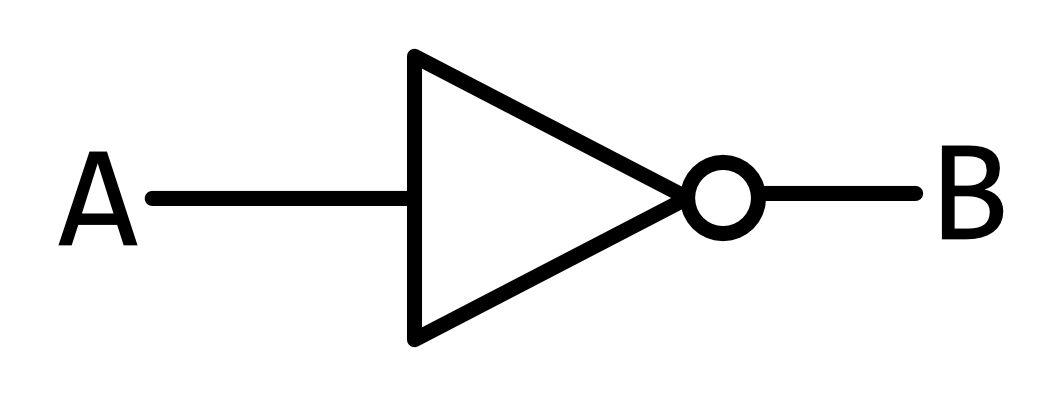Below is an “AND gate,” with two inputs, A and B, and one output, C. If both inputs are 1, then the output is 1. If either or both inputs are 0, then the output is 0.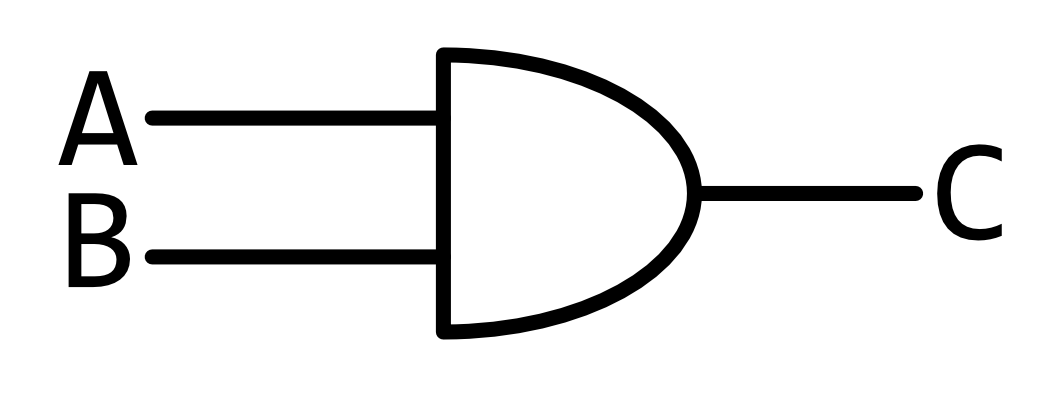Below is an “OR gate,” with two inputs, A and B, and one output, C. If either or both inputs are 1, then the output is 1. If both inputs are 0, then the output is 0.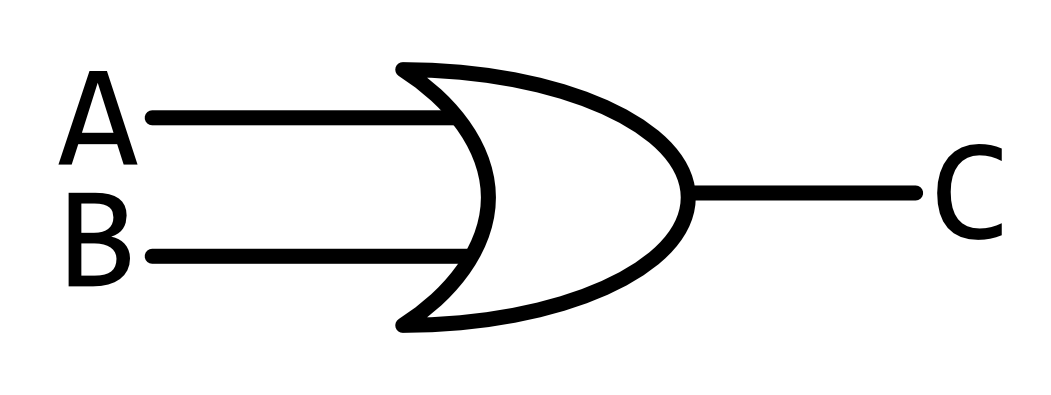These logic gates can be combined to form more-complex circuits. For example, consider the circuit below, with two inputs, A and B. This circuit inverts A (but not B) via a NOT gate, passing its output and B into an AND gate, the output of which is C. As a result, C will be 1 if, and only if, A is 0 and B is 1; otherwise, C will be 0.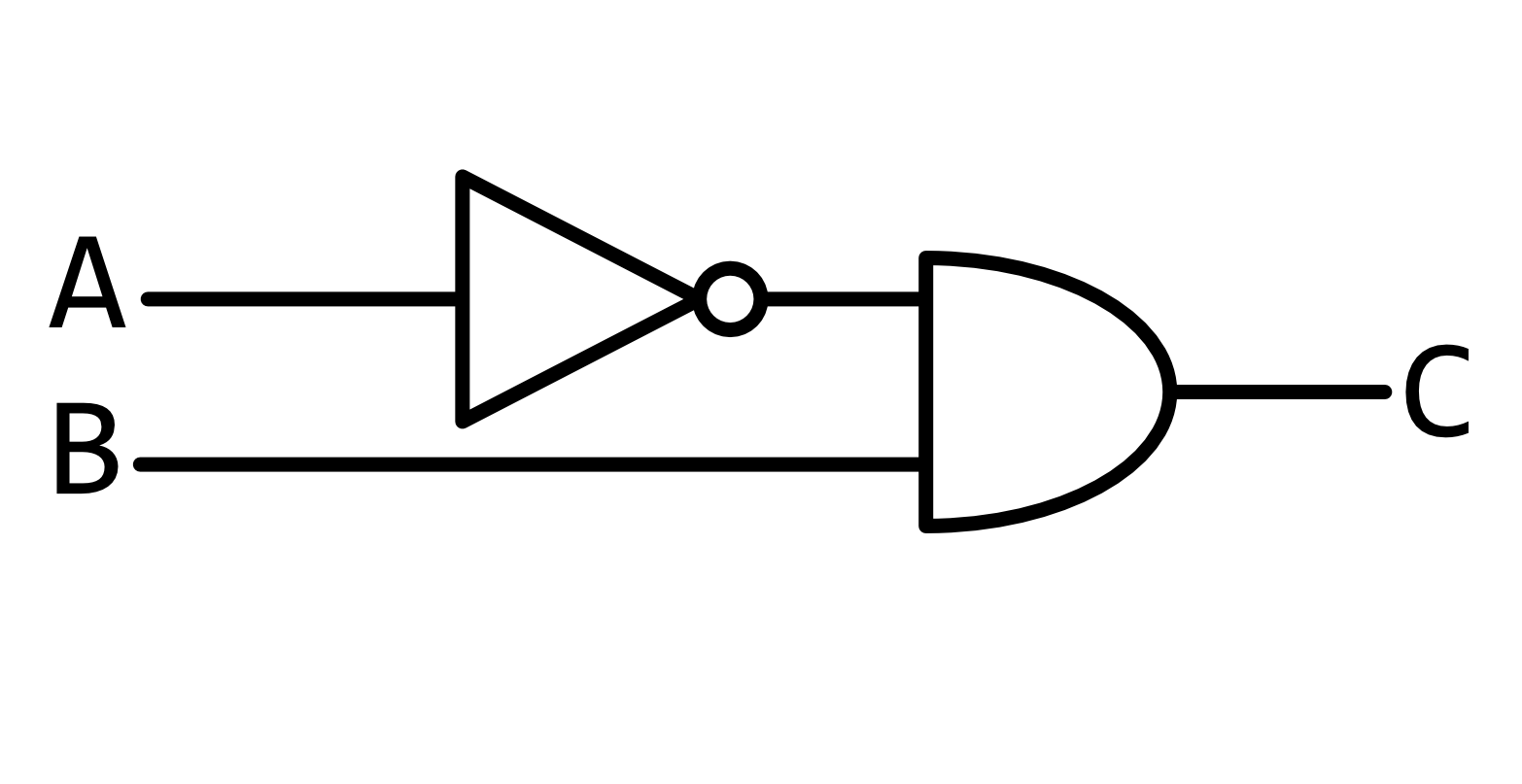1. (2 points.) Consider the circuit below. In no more than three sentences, under what circumstances would the circuit output 1?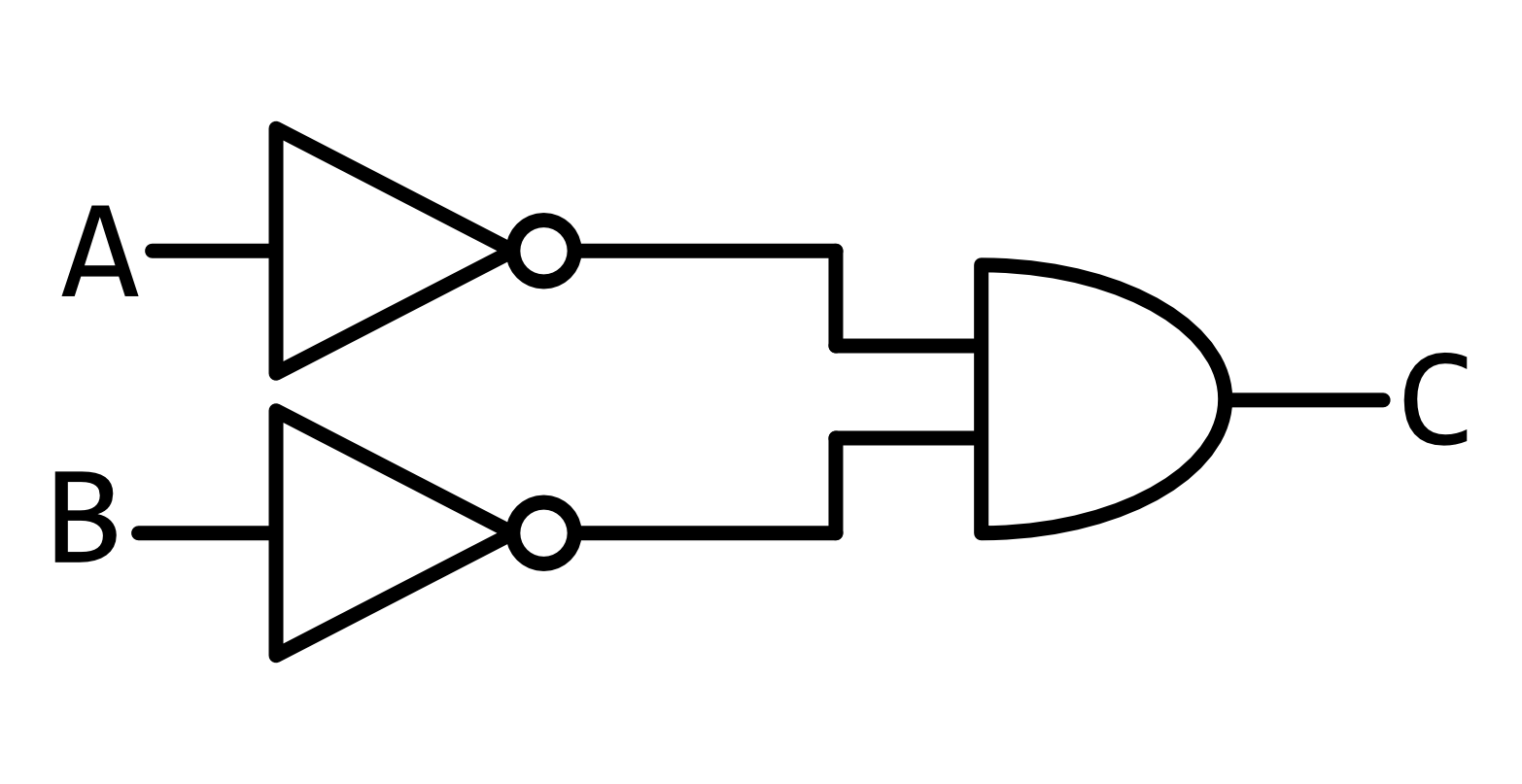2. (3 points.) Consider the circuit below. In no more than three sentences, under what circumstances would the circuit output 1? Note that A splits into two wires: whatever its value is propagates to both wires. The same goes for B. Also note that the curved line in the circuit represents a wire that travels over or under the horizontal wire, without actually touching it.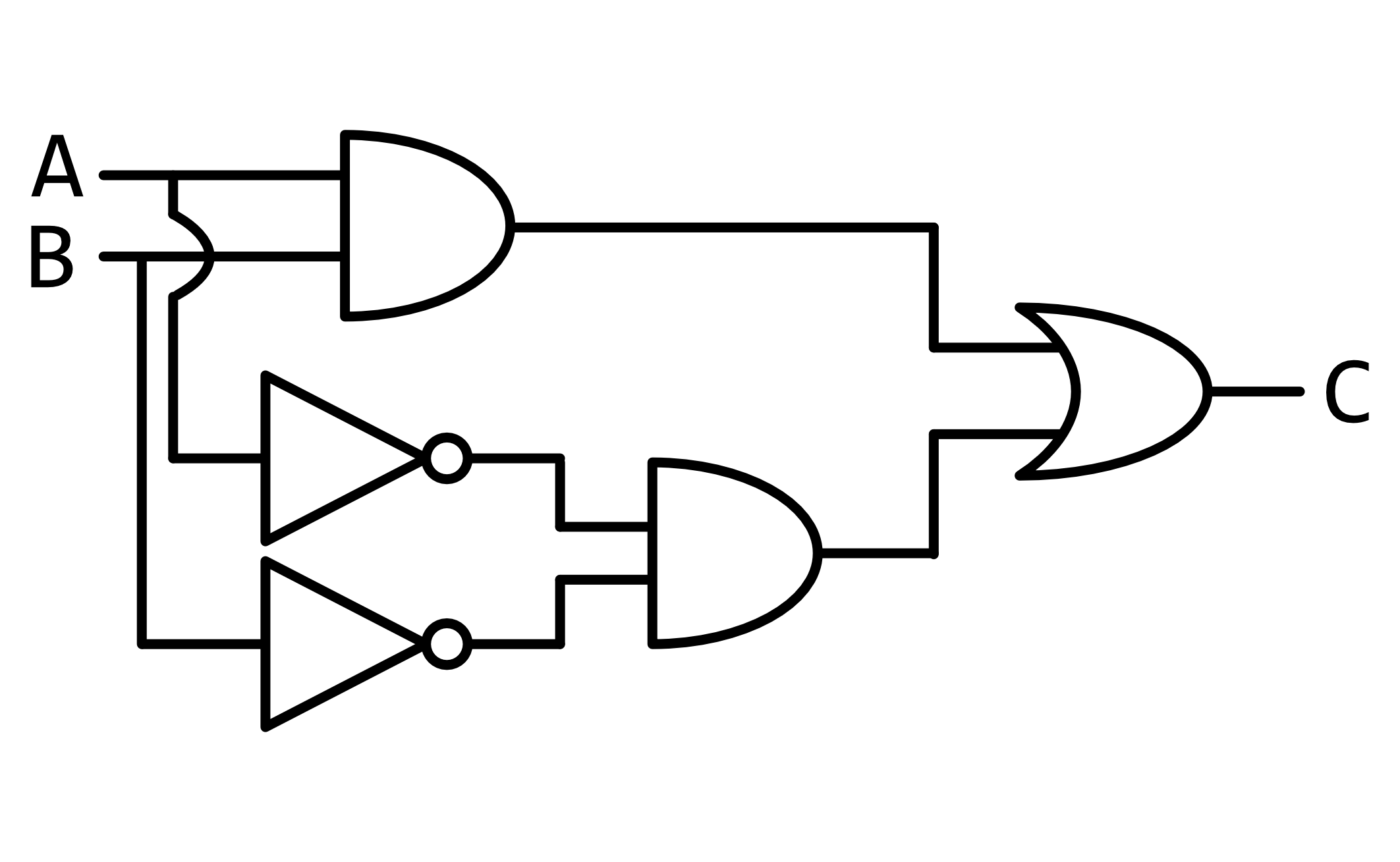3. (3 points.) Consider the circuit below. In no more than three sentences, under what circumstances would the circuit output 1? Note that A splits into two wires: whatever its value is propagates to both wires. The same goes for B. Also note that the curved lines in the circuit represent wires that travel over or under the horizontal wires, without actually touching them.